## Sunday, August 5, 2007

### Irodov Problem 1.63(a) The force diagram is depicted in the figure beside. Suppose that the massaccelerates downwards at a rate w.

Force Diagram of: There are two forces acting - the tension in the string T pulling the mass up and the gravitational force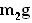pulling it downwards. Thus, we have,Force Diagram of: We shall resolve the forces acting on this mass along parallel and perpendicular directions to the incline.

In the direction perpendicular to the incline there are two forces acting on the body, the normal reaction N and the component of force of gravity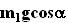. There is no component of acceleration for the mass along the perpendicular direction to the incline.
Thus, we have,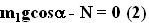Now considering forces along the direction parallel to the incline. There are three forces acting on the body - the gravitational forcepulling the mass downwards, the tension T in the string pulling the block upwards and the force of friction Nk that opposes the motion of the body. Since the mass moves up along the incline in this part, the force of friction will act in the downward slope direction. Thus we have,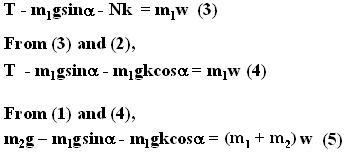Since w is a positive quantity for the mass to move down, the condition for the mass to move up is given by,(b) The force diagram for the case when the massis moving up is also provided in the figure. The only difference between the two cases is that the direction of force of friction acting onchanges its direction (since the direction of motion is now changed as well) and is now direction towards the upward slope. Thus, for this case the equivalent of equation (5) becomes,In (7), w is the acceleration of the massalong the downward slope direction. Now, since w>0 we have,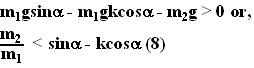(c) The body will be at rest when none of the conditions (6) and (8) are satisfied and is given by,